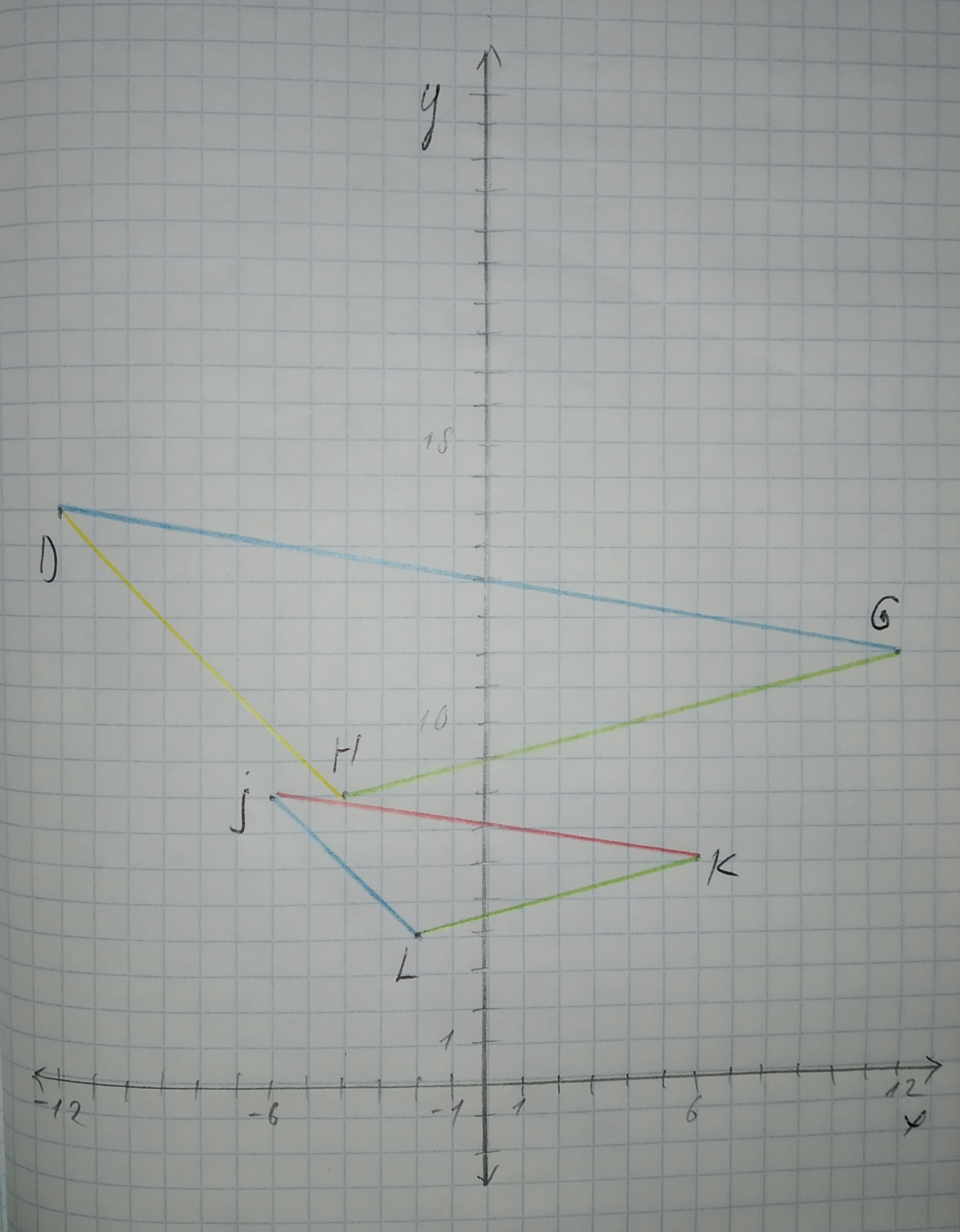# Draw a graph for the original figure and its dilated image. Check whether the dilation is a similarity transformation or not. Given: The given vertices are J(-6,8),K(6,6),L(-2,4),D(-12,16),G(12,12),H(-4,8)foass77W 2021-02-13 Answered
Draw a graph for the original figure and its dilated image. Check whether the dilation is a similarity transformation or not.
Given:
The given vertices are
J(-6,8),K(6,6),L(-2,4),D(-12,16),G(12,12),H(-4,8)
You can still ask an expert for help

• Questions are typically answered in as fast as 30 minutes

Solve your problem for the price of one coffee

• Math expert for every subject
• Pay only if we can solve itpattererX

Calculation:
The graph for the given points J(-6,8),K(6,6),L(-2,4),D(-12,16),G(12,12),H(-4,8) is given below.Find the distance(using distance formula)of corresponding sides and find the ratio.
$DH=\sqrt{{\left[-12-\left(-4\right)\right]}^{2}+{\left[16-8\right]}^{2}}=\sqrt{128}=8\sqrt{2}$
$JL=\sqrt{{\left[-6-\left(-2\right)\right]}^{2}+{\left(8-4\right)}^{2}}=\sqrt{32}=4\sqrt{2}$
$\frac{DH}{JL}=\frac{8\sqrt{2}}{4\sqrt{2}}=2$
$GH=\sqrt{{\left[-12-\left(-4\right)\right]}^{2}+{\left(12-8\right)}^{2}}=\sqrt{272}=4\sqrt{17}$
$KL=\sqrt{{\left[6-\left(-2\right)\right]}^{2}+{\left(6-4\right)}^{2}}=\sqrt{68}=2\sqrt{17}$
$\frac{GH}{KL}=\frac{4\sqrt{17}}{2\sqrt{17}}=2$
$DG={\sqrt{\left[-12-12\right)}}^{2}+{\left(16-12\right)}^{2}\right)=\sqrt{592}=4\sqrt{37}$
$JK=\sqrt{{\left[-6-6\right]}^{2}+{\left(8-6\right)}^{2}}=\sqrt{148}=2\sqrt{37}$
$\frac{DG}{JK}=\frac{4\sqrt{37}}{2\sqrt{37}}=2$
From the above result, the lenght of the sides are proportional and the angles between them are congruent.
By the use of SSS similarity,
$\mathrm{△}JLK\sim \mathrm{△}DHG$.
Hence the dilation is a similarity transformation.